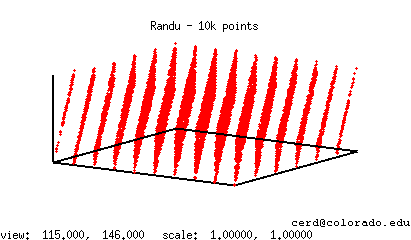Description: Code that generates pseudo-random numbers using Randu, a multiplicative linear congruential pseudo-random number generator.

Randu is a pseudo-random number generator dating back to the 1960s that was included in IBM's scientific subroutine package for System/360 mainframe computer systems. It generates successive random numbers by using the following recurrence:

ri+1=65539 * ri modulo 231

Here, the initial value, r0, is known as the random seed.

Note that, due to the choice of the multiplier and modulus, this algorithm can be implemented using just a handful of add and bit manipulation operations (see the C, C++, or Java implementations below). This was intentional as it allowed for a particularly efficient implementation on early computer systems. However, it later fell out of favor due to a number of undesirable properties in the sequence of values it generated that can be attributed to its given multiplier and modulus. For instance, if the coordinates for a large number of 3-dimensional points are generated using Randu, all of the points will be found to lie along 15 planes. This phenomena is shown below in a scatter plot of 10,000 Randu generated points. Ideally, such pseudo-randomly generated points should fairly uniformly fill the cube, as would happen if a set of truly random points were used.Additionally, this generator lacks a full period. That is, random number generators defined by a recurrence like the one above will eventually produce a value that was previously seen earlier on in the sequence. When this happens, the subsequent sequence of values generated will be identical to the sequence previously generated after the repeated value. For example, if Randu is started with a random seed of 1 and is allowed to run indefinitely, then it will generate a sequence like the following:

65539, 393225, 1769499, 7077969, 26542323.....1, 65539, 393225, 1769499, 7077969, 26542323..... 1, 65539, 393225, 1769499, 7077969, 26542323.....

The period of a random number generator is the number of values it outputs prior to repeating itself. A generator that produces values modulo some value m, is seen to have a full period if the period length is equal to m. Put another way, a generator has a full period if it produces all integer values in the range [0,m). Randu's maxium period length is only 229, which is observed whenever Randu is started with an odd random seed. Thus, it can only generate a forth of the values in the range [0,231).

Finally, in many of the code samples below, the state of the generator is stored as a global variable. While the use of global variables is generally undesirable, this matches the implementation of random number generators in many older standard libraries.

Languages: C, C++, Java, Perl, Python, Ruby, Octave(/Matlab like), Scheme, and Bash shell script.